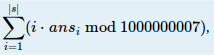odd doctor 已更新

# Easy Sequence

Time Limit
2s
Memory Limit
131072KB
Judge Program
Standard
Ratio(Solve/Submit)
50.00%(1/2)
Description:

soda has a string containing only two characters -- '(' and ')'. For every character in the string, soda wants to know the number of valid substrings which contain that character.

Note:
An empty string is valid. If S is valid, (S) is valid. If U,V are valid, UV is valid.

Input:

There are multiple test cases. The first line of input contains an integer T, indicating the number of test cases. For each test case:

A string s consisting of '(' or ')' (1|s|106).

Output:

For each test case, output an integer m=where ansi is the number of valid substrings which contain i-th character.

Sample Input:
2
()()
((()))
Sample Output:
20
42
Hint:

For the second case, ans={1,2,3,3,2,1}, then m=1⋅1+2⋅2+3⋅3+4⋅3+5⋅2+6⋅1=42

Source:

2015 Multi-University Training Contest 6

Submit